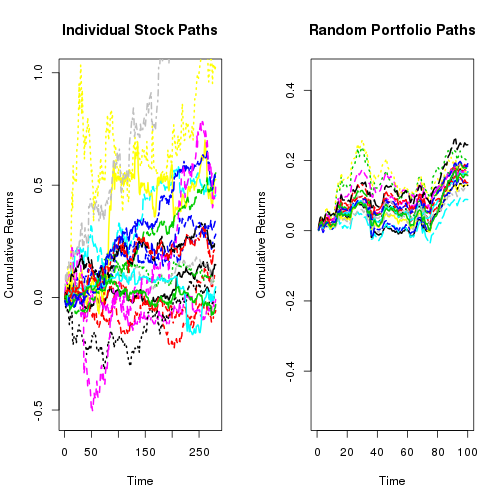# Naive Diversification

The code below will fetch returns for the 20 stocks listed, and plot each stock's cumulative return. The mode will then generate 20 random portfolios of these 20 stocks, and plots each of the 20 portfolios. Ultimately, you can nicely see the effect of completely naive diversification. Markowitz improves on this by diversifying in an optimal way.

``````library(quantmod)
env <- new.env()
Symbols <- c("BP", "RHT", "VZ", "JPM", "CHK", "ECA", "AMD", "MO", "AAPL", "IBM",
"LMT", "BA", "EGAS", "DDD", "CIMT", "P", "GS", "C", "T", "MSFT")
getSymbols(Symbols = Symbols, env = env, from = "2013-01-01")

args <- eapply(env = env, FUN = function(x) {
ClCl(x)
})[Symbols]
returns <- na.omit(do.call(what = merge, args = args))
colnames(returns) <- Symbols

srets <- as.matrix(returns)
par(mfrow = c(1, 2))
plot(cumsum(srets[, 1]), ylim = c(-0.53, 1), lwd = 2, lty = 1, col = 1, type = "l",
main = "Individual Stock Paths", ylab = "Cumulative Returns", xlab = "Time")
for (i in 2:20) {
Sys.sleep(2)
lines(cumsum(srets[, i]), lwd = 2, lty = i, col = i, type = "l")
}

## Create Weights ##;
W <- matrix(0, nrow = 20, ncol = 20)
for (i in 1:20) {
W[i, ] <- 1/sample(5:40, 20, replace = FALSE)
}

## Weights sum to 1 ##;

for (i in 1:20) {
W[i, 20] <- 1 - sum(W[i, 1:19])
}

b <- matrix(0, nrow = 100, ncol = 20)

## Plot Random Portfolios

msrets <- as.matrix(srets)
plot(5000, ylim = c(-0.53, 0.45), xlim = c(0, 100), lwd = 2, lty = 1, col = 1,
type = "l", main = "Random Portfolio Paths", ylab = "Cumulative Returns",
xlab = "Time")
for (j in 1:20) {
Sys.sleep(2)
for (i in 1:100) {
b[i, j] <- t(msrets[i, ]) %*% W[j, ]
}
lines(cumsum(b[, j]), lwd = 2, lty = j, col = j, type = "l")
}
``````